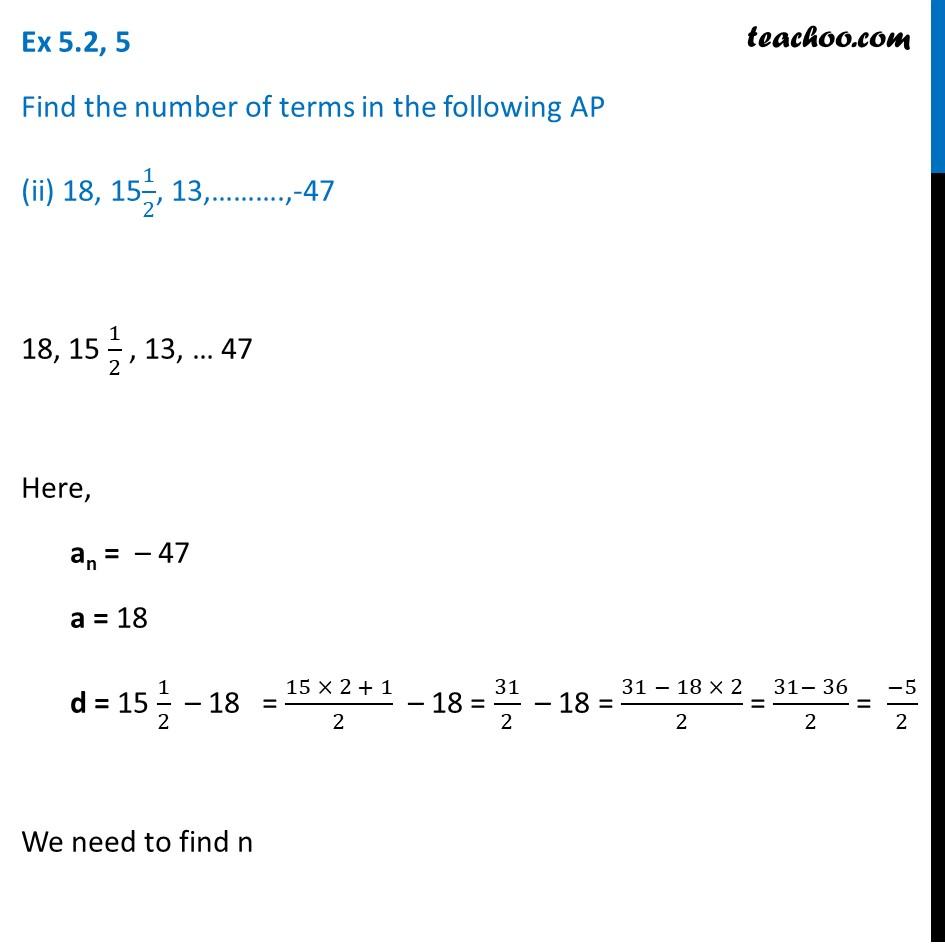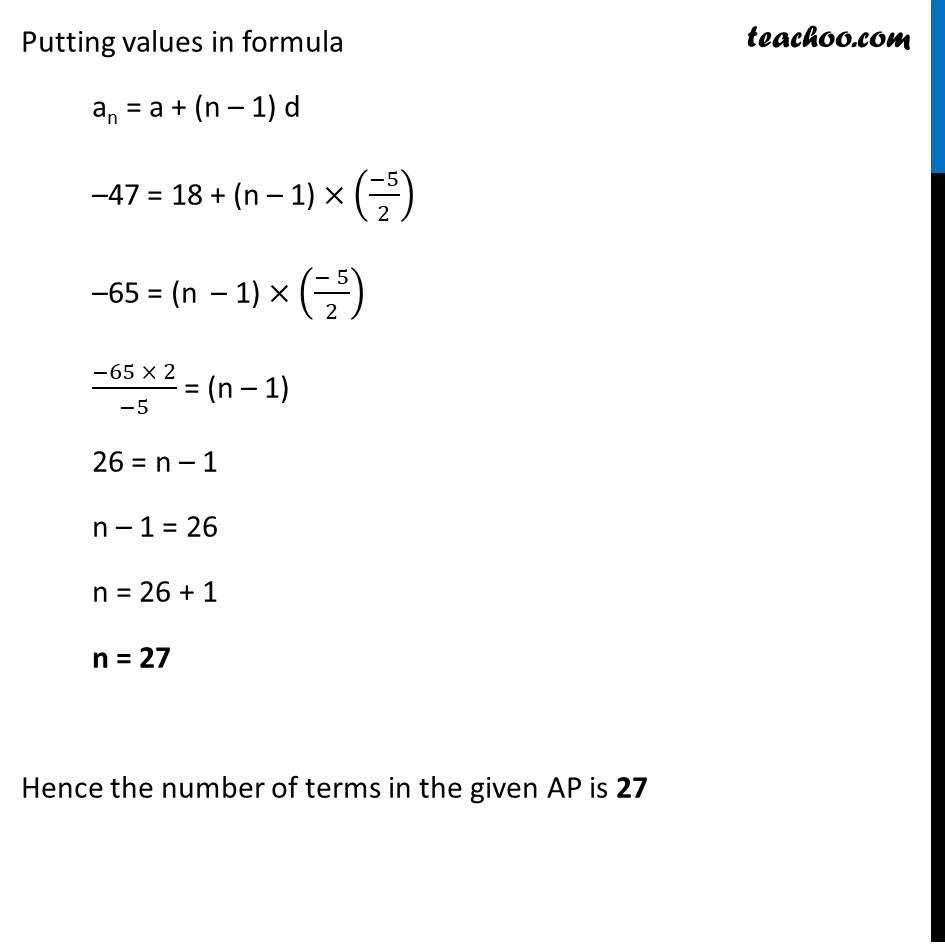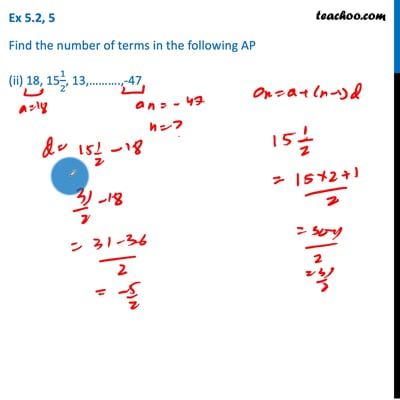Ex 5.2

Chapter 5 Class 10 Arithmetic Progressions
Serial order wiseThis video is only available for Teachoo black users

Introducing your new favourite teacher - Teachoo Black, at only ₹83 per month

### Transcript

Ex 5.2, 5 Find the number of terms in the following AP (ii) 18, 151/2, 13,……….,-47 18, 15 1/2 , 13, … 47 Here, an = – 47 a = 18 d = 15 1/2 – 18 = (15 × 2 + 1)/2 – 18 = 31/2 – 18 = (31 − 18 × 2)/2 = (31− 36)/2 = (−5)/2 We need to find n Putting values in formula an = a + (n – 1) d –47 = 18 + (n – 1) × ((−5)/2) –65 = (n – 1) × ((− 5)/2) (−65 × 2)/(−5) = (n – 1) 26 = n – 1 n – 1 = 26 n = 26 + 1 n = 27 Hence the number of terms in the given AP is 27